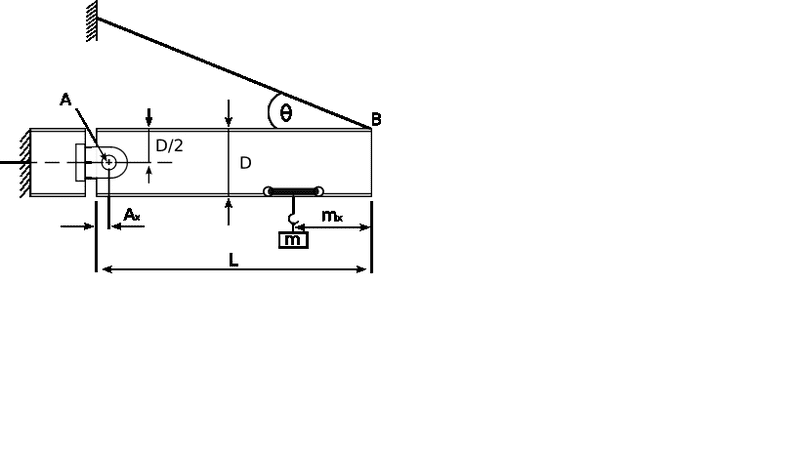# Tension in a cable supporting a hinged beam

I have tried to complete the following question by taking moments about point A, but to no avail. Could anybody help me with where i am going wrong. Do I need to include perpendicular distance?

The I-beam A-B shown below is supported by a cable at B and a pin at A. The cable is inclined at an angle, θ = 21° to the longitudinal axis of the beam. The beam supports a load, m, of 12 kN at a distance mx = 1.5 m from B.If the mass of the beam is 95 kg/m and its total weight can be assumed to act through the centre of gravity of the beam, calculate:

a) the cable tension, T

b) the resultant force acting on the pin at A.

Given:

Ax = 0.12 m, Beam Depth, D = 0.5 m, Beam Length, L = 5 m, Gravity, g = 9.81 m/s2

Part a)

Weight of beam = 4.65975 kN

By taking moments about A, T = 26.09±0.2 kN

Part b)

To determine the resultant at A, calculate the horizontal and vertical reactions AH and AV respectively:

AH = 24.36±0.2 kN

AV = 7.31±0.2 kN

Therefore, the resultant (using Pythagoras), R = 25.43±0.2 kN
Incorrect

Here is my solution to the cable tension

(4.88)(T)(sin21)+(111.834x0.06)-(4.5479x10^3)(2.44)-(12x10^3)(3.38)=0

1.7488(T)=+6.7098+11.0968x10^3+40.56x10^3

1.7488(T)=51650.0902

(T)= (51.65x10^3)/1.7488 =29.53kn

Simon Bridge